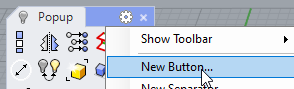# Round off scale/size and position values in multiple objects

Hello everyone.

I’m new in Rhino.
I need to automatically round scale/size and position of selected objects.
Using a script would be great, but I don’t know how to develop scripts.

Currently I make these modifications using BoxEdit one object at a time.
But it is very difficult in projects with hundreds of geometries.
So I need to round the scale and position automatically.

Before:

After:

It can surely be done with rhinoscript, but doing this with grasshopper is much more easier, simpler and faster “to code”.
Would it be the same for you?
(Grasshopper output will not retain original geometry properties like colour, layer, material, etc etc etc…)

Hello Riccardo. Thanks for answering me.
With grasshopper it wouldn’t work for me.With rhinoscript it would be perfect. But I don’t know how to develop scripts.

I’m new in Rhino
I don’t know how I could do it in Grasshopper or rhinoscript.

What are the rules? Always rounding to the Floor? and being changed from the bounding box center?``````-runscript (
RoundBoxEdit

Sub RoundBoxEdit
objs = Rhino.GetObjects("Select objects",0)
If IsArray(objs) Then
For Each obj In objs
RoundOneBoxEdit obj
Next
ElseIf Not IsNull(objs) Then
RoundOneBoxEdit objs
End If
End Sub

Sub RoundOneBoxEdit(object)
box = Rhino.BoundingBox(object)
p0 = box(0)
p6 = box(6)
c = Array((p0(0)+p6(0))/2,(p0(1)+p6(1))/2,(p0(2)+p6(2))/2)
rc = Array(Round(c(0)),Round(c(1)),Round(c(2)))
v = Rhino.VectorCreate(rc,c)
x = box(6)(0)-c(0)
y = box(6)(1)-c(1)
z = box(6)(2)-c(2)
scaleX=ScaleToRoundFactor(x)
scaleY=ScaleToRoundFactor(y)
scaleZ=ScaleToRoundFactor(z)
Rhino.MoveObject object , v
Rhino.ScaleObject object , rc , Array(scaleX , scaleY , scaleZ)
End Sub

Function ScaleToRoundFactor(num)
If num > 0.5 Then
ScaleToRoundFactor = Round(num)/num
Else
ScaleToRoundFactor = 0
End If
End Function

)
``````

Oh, I did a mistake.
The script was working at the min,min,min as center “Pivot location”.
Corrected the code to work for the cen,cen,cen …

Thank you , @jeremy5 , for pointing this out!

1 Like

Riccardo. Thank you very much!
You saved my life.
I really needed this solution.
It’s working perfectly so far.
Thanks for helping me.

1 Like